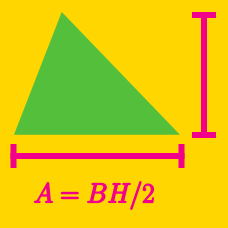Geometry

# Area of Triangles: Level 1 Challenges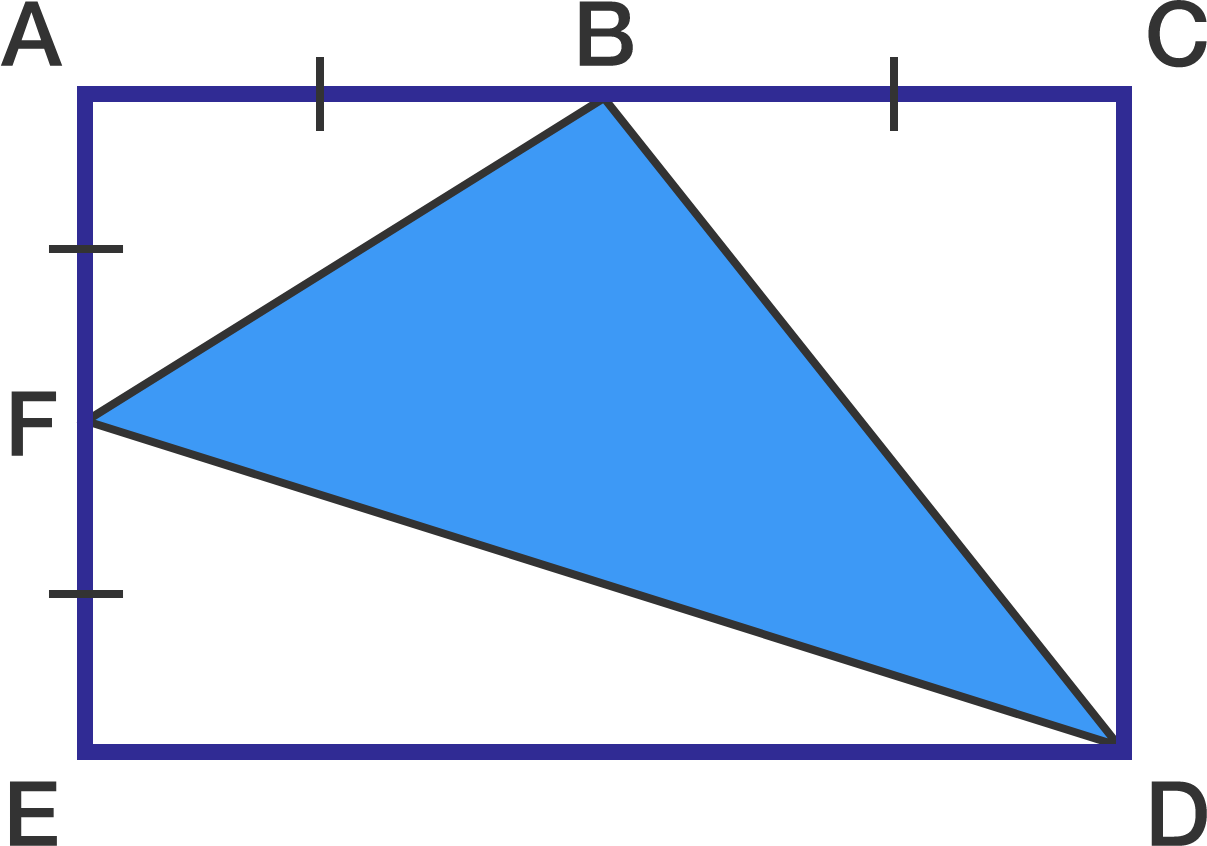The rectangle in the diagram has an area equal to 640 $\text{cm}^2$.

Points B and F are midpoints of sides AC and AE, respectively.

What is the area of triangle BDF in $\text{cm}^2?$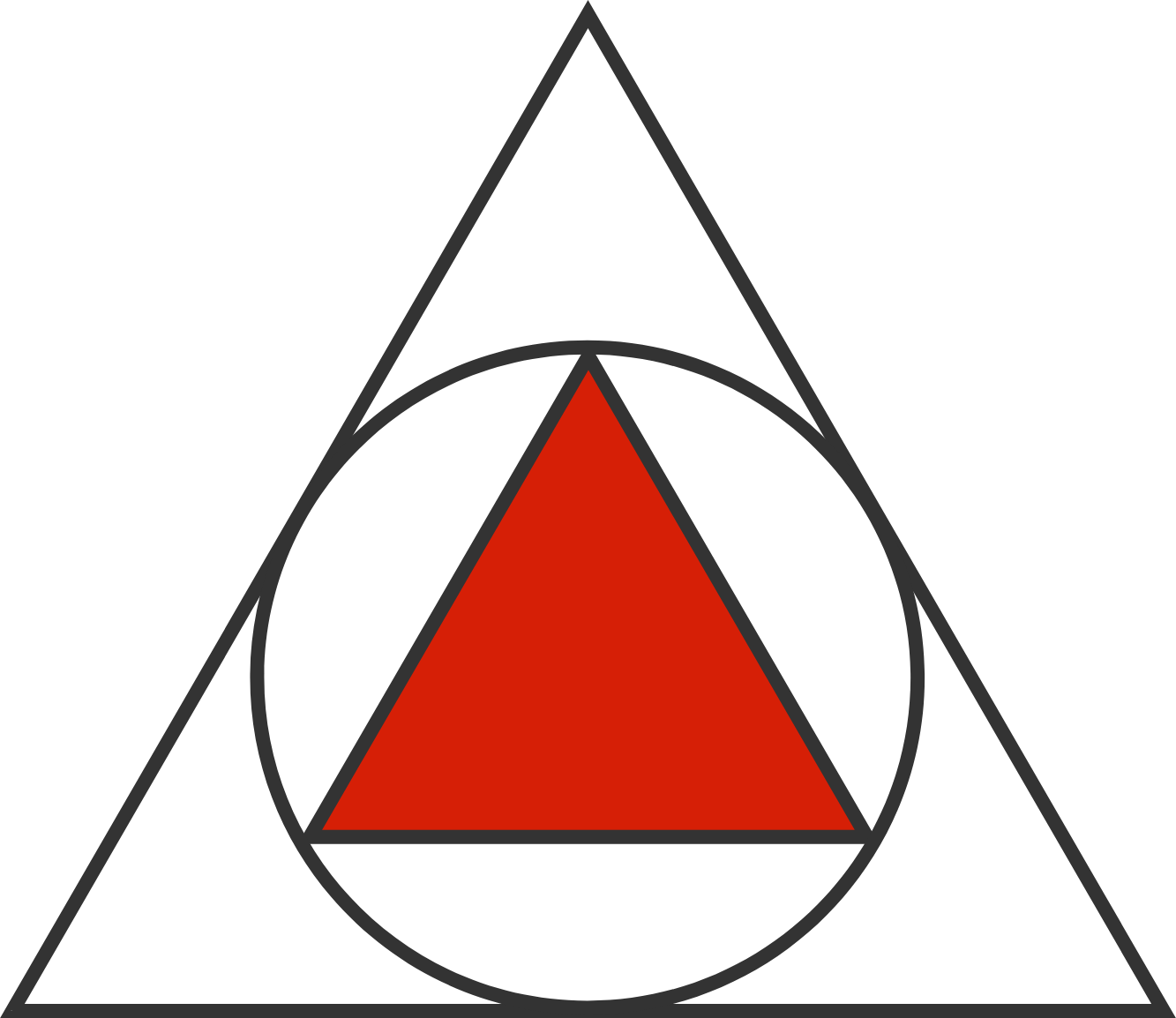In a large equilateral triangle, we draw the incircle. We then draw a smaller equilateral triangle in the incircle.

What is the ratio of the area of the smaller equilateral triangle to that of the larger equilateral triangle?

A triangle has sides 15, 41, and 52. What is its area?

$\triangle ABC$ is a right triangle and line segment $AD$ is an altitude.
If $AB = 10$ and $BD = 8$, then what is the area of $\triangle ABC$?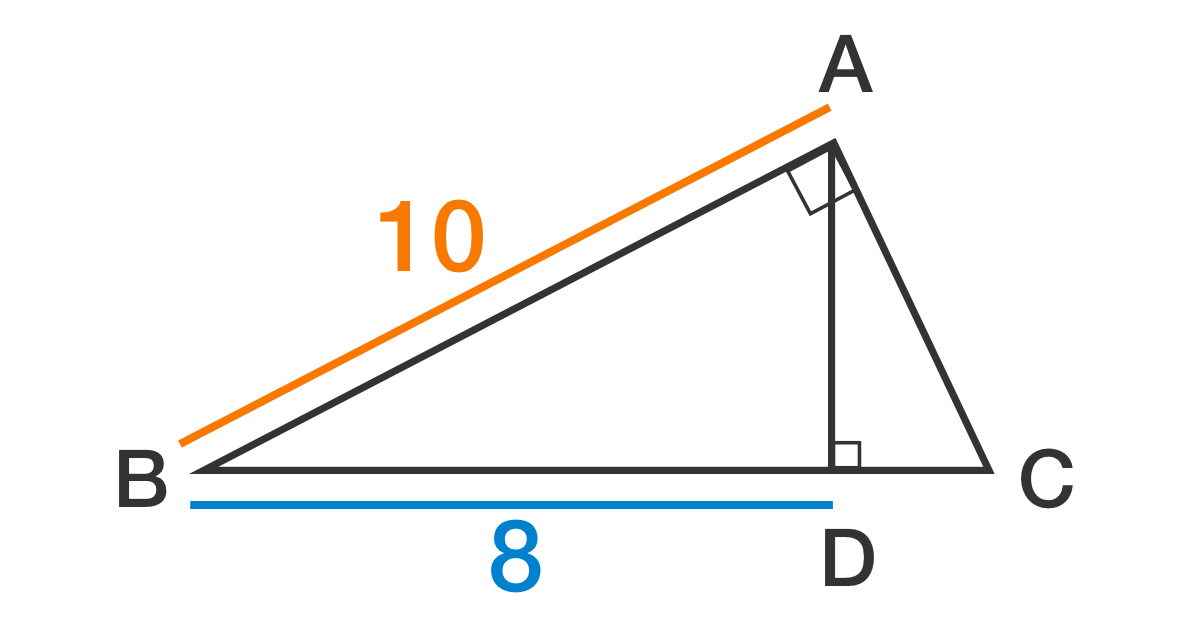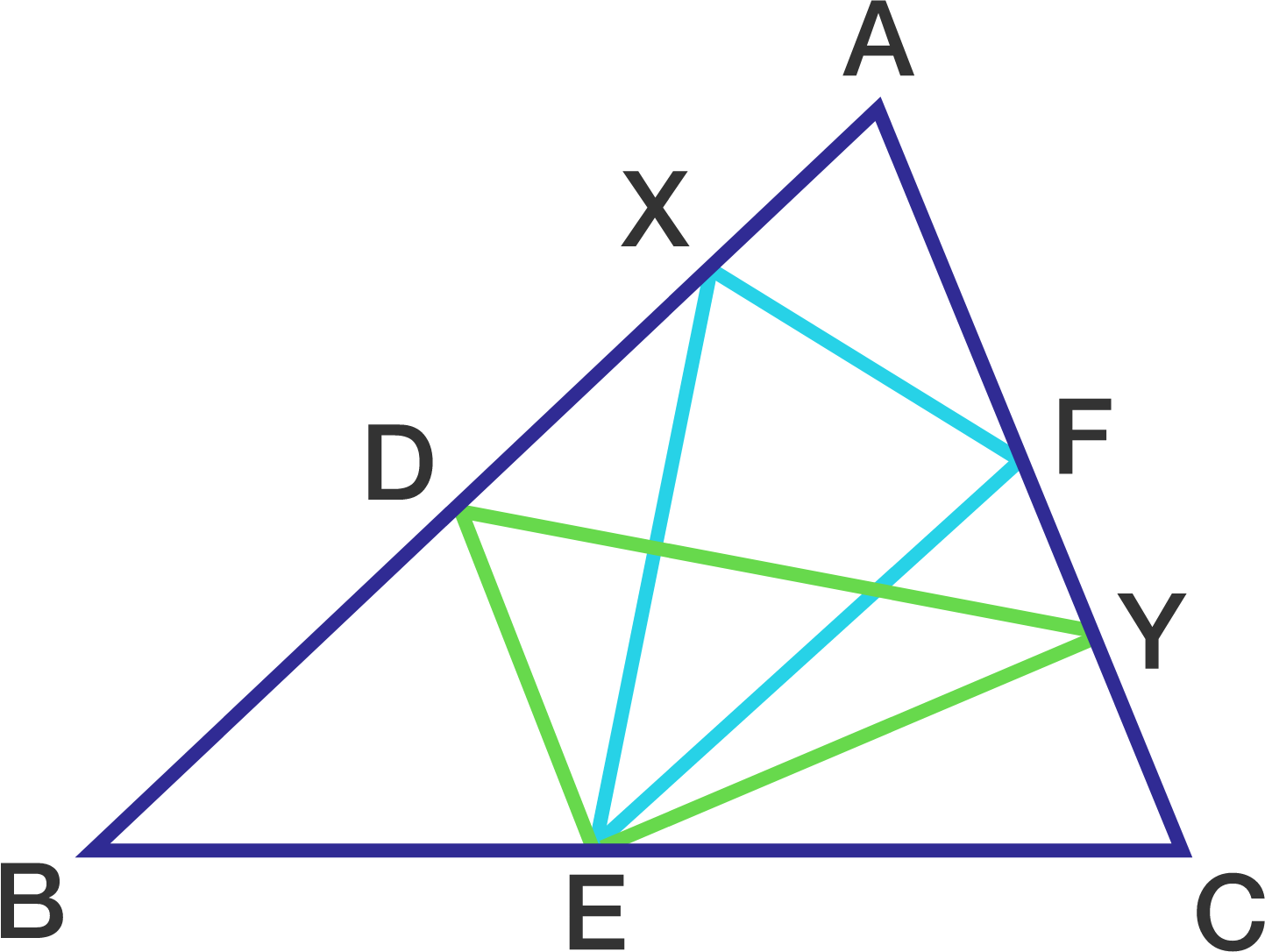The diagram above shows a triangle, it is known that $ADEF$ is a parallelogram, $X$ is a point on line $AB$, $Y$ is a point on line $AC$. If the area of $\triangle XEF$ is 1, then the area of $\triangle DEY$ is...

×